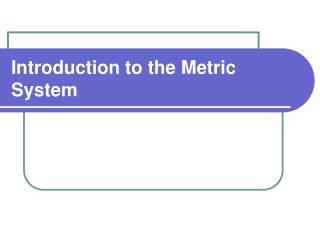Download PresentationIntroduction to the Metric System

# Introduction to the Metric System - PowerPoint PPT PresentationDownload Presentation## Introduction to the Metric System

- - - - - - - - - - - - - - - - - - - - - - - - - - - E N D - - - - - - - - - - - - - - - - - - - - - - - - - - -
##### Presentation Transcript

1. Introduction to the Metric System

2. History • Called Systeme International d’Unitès, • or SI - International System of Units • Revised periodically • by International Bureau of Weight and Measures

3. Customary Units of Measurement • The English System • a collection of functionally unrelated units • Difficult to convert from one unit to another • Ex. 1 ft = 12 inches = 0.33 yard = 1/5280 miles • Customary Units • length - inch, foot, yard, mile • weight/mass - ounce, pound • volume - teaspoon, cup, quart, gallon • temperature - degrees Fahrenheit , KELVIN • time - minutes, hours

4. Advantages of Using the Metric System • Universal - used everywhere • by all scientists to communicate • by all industrialized nations • except United States • U.S. loses billions of dollars in trade

5. Advantages of Using the Metric System • Simple to use • A few base unitsmake up all measurements • length - meter • mass - grams • volume - liters • temperature – degrees Celsius • time - seconds

6. Advantages of Using the Metric System • There is only one unit of measurement for each type of quantity • To simplify things, very small and very large numbers are expressed as multiples of the base unit. • Prefixes are used to represent how much smaller or larger the quantity is compared to the base unit. • Easy toconvert from one unit to another • shift decimal point right • shift decimal point left

7. Advantages of Using the Metric System • Same set of prefixes for all units • Greek - multiples of the base • kilo -1000 × the base • hecto - 100 × the base • deka - 10 × the base • Latin - fractions of the base • deci - tenths of the base • centi -hundredths of the base • milli - thousandths of the base

8. Metric Prefixes

9. Units of Length • Length - the distance between two points • standard unit is meter(m) • long distances are measured in km • Measured using a meter stick or ruler

10. Prefixes and Units of Length • centimeter - cm • 1 m = 100 cm • 1 cm = 1/100th m • millimeter - mm • 1 m = 1000 mm • 1 mm = 1/1000th m • 10 mm = 1 cm • measures very small lengths • kilometer - km • 1 km = 1000 m • 1 m = 1/1000th km • measures long distances

11. Measuring Mass • Mass - the quantity of matter in an object • standard unit is gram(g) • Measured using a digital scale or triple beam balance

12. Measuring Volume and Capacity • Volume - the amount of space occupied by an object • standard unit is liter(L) • 1 L = 1000 ml = 1000 cm3 = 1 dm3 • Measured using a graduated cylinder • Capacity - a measure of the volume inside a container

13. Prefixes and Units of VolumeSTOP HERE • Liter - L • 1 L = 1000 milliliters • 1 L = 1000 cubic centimeters = 1000 cm3 • milliliter - mL • measures small volumes • 1 mL = 1 cubic centimeter • 1000 mL = 1 Liter • 1 mL = 1/1000th liter • kiloliter - kL • measures large volumes • 1 kL = 1000 L

14. Unit Conversion Practice • Example:Convert 4 ounces to kilograms.